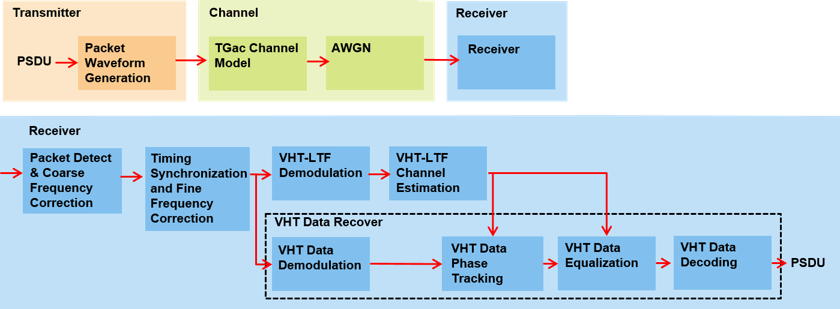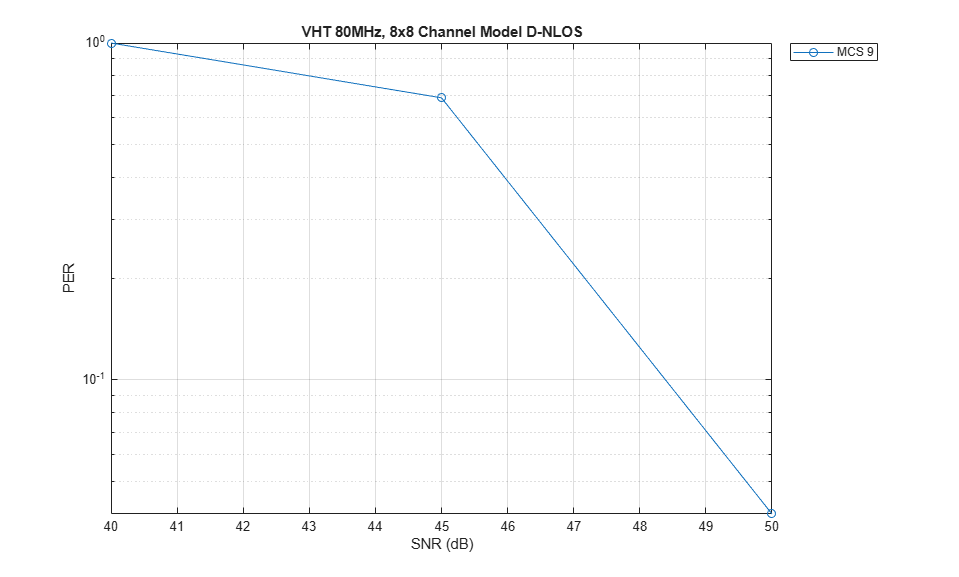# 802.11ac Packet Error Rate Simulation for 8x8 TGac Channel

This example shows how to measure the packet error rate of an IEEE® 802.11ac™ VHT link using an end-to-end simulation with a fading TGac channel model and additive white Gaussian noise.

### Introduction

In this example an end-to-end simulation is used to determine the packet error rate for an 802.11ac [ 1 ] VHT link with a fading channel at a selection of SNR points. At each SNR point multiple packets are transmitted through a channel, demodulated and the PSDUs recovered. The PSDUs are compared to those transmitted to determine the number of packet errors and hence the packet error rate. Packet detection, timing synchronization, carrier frequency offset correction and phase tracking are performed by the receiver. The processing for each packet is summarized in the followingThis example also demonstrates how a `parfor` loop can be used instead of the `for` loop when simulating each SNR point to speed up a simulation. The `parfor` function, as part of the Parallel Computing Toolbox™, executes processing for each SNR in parallel to reduce the total simulation time.

### Waveform Configuration

An 802.11ac VHT transmission is simulated in this example. The VHT format configuration object, `wlanVHTConfig`, contains the format-specific configuration of the transmission. The properties of the object contain the configuration. In this example the object is configured for a 80 MHz channel bandwidth, 8 transmit antennas, 8 space-time streams, no space time block coding, 256-QAM rate-5/6 (MCS 9), and binary convolutional coding (BCC).

```% Create a format configuration object for a 8-by-8 VHT transmission cfgVHT = wlanVHTConfig; cfgVHT.ChannelBandwidth = 'CBW80'; % 80 MHz channel bandwidth cfgVHT.NumTransmitAntennas = 8; % 8 transmit antennas cfgVHT.NumSpaceTimeStreams = 8; % 8 space-time streams cfgVHT.APEPLength = 3000; % APEP length in bytes cfgVHT.MCS = 9; % 256-QAM rate-5/6 cfgVHT.ChannelCoding = 'BCC'; % Binary convolutional coding ```

### Channel Configuration

In this example a TGac N-LOS channel model is used with delay profile Model-D. For Model-D when the distance between transmitter and receiver is greater than or equal to 10 meters, the model is NLOS. This is described further in `wlanTGacChannel`. An 8x8 MIMO channel is simulated in this example therefore 8 receive antennas are specified.

```% Create and configure the channel tgacChannel = wlanTGacChannel; tgacChannel.DelayProfile = 'Model-D'; tgacChannel.NumReceiveAntennas = 8; tgacChannel.TransmitReceiveDistance = 10; % Distance in meters for NLOS tgacChannel.ChannelBandwidth = cfgVHT.ChannelBandwidth; tgacChannel.NumTransmitAntennas = cfgVHT.NumTransmitAntennas; tgacChannel.LargeScaleFadingEffect = 'None'; tgacChannel.NormalizeChannelOutputs = false; ```

### Simulation Parameters

For each SNR point in the vector `snr` a number of packets are generated, passed through a channel and demodulated to determine the packet error rate.

```snr = 40:5:50; ```

The number of packets tested at each SNR point is controlled by two parameters:

1. `maxNumErrors` is the maximum number of packet errors simulated at each SNR point. When the number of packet errors reaches this limit, the simulation at this SNR point is complete.

2. `maxNumPackets` is the maximum number of packets simulated at each SNR point and limits the length of the simulation if the packet error limit is not reached.

The numbers chosen in this example will lead to a very short simulation. For meaningful results we recommend increasing the numbers.

```maxNumErrors = 10; % The maximum number of packet errors at an SNR point maxNumPackets = 100; % The maximum number of packets at an SNR point ```

Set the remaining variables for the simulation.

```% Get the baseband sampling rate fs = wlanSampleRate(cfgVHT); % Get the OFDM info ofdmInfo = wlanVHTOFDMInfo('VHT-Data',cfgVHT); % Set the sampling rate of the channel tgacChannel.SampleRate = fs; % Indices for accessing each field within the time-domain packet ind = wlanFieldIndices(cfgVHT); ```

### Processing SNR Points

For each SNR point a number of packets are tested and the packet error rate calculated.

For each packet the following processing steps occur:

1. A PSDU is created and encoded to create a single packet waveform.

2. The waveform is passed through a different realization of the TGac channel model.

3. AWGN is added to the received waveform to create the desired average SNR per active subcarrier after OFDM demodulation.

4. The packet is detected.

5. Coarse carrier frequency offset is estimated and corrected.

6. Fine timing synchronization is established. The L-STF, L-LTF and L-SIG samples are provided for fine timing to allow for packet detection at the start or end of the L-STF.

7. Fine carrier frequency offset is estimated and corrected.

8. The VHT-LTF is extracted from the synchronized received waveform. The VHT-LTF is OFDM demodulated and channel estimation is performed.

9. The VHT Data field is extracted from the synchronized received waveform. The PSDU is recovered using the extracted field and the channel estimate.

A `parfor` loop can be used to parallelize processing of the SNR points. To enable the use of parallel computing for increased speed comment out the `for` statement and uncomment the `parfor` statement below.

```S = numel(snr); packetErrorRate = zeros(S,1); %parfor i = 1:S % Use 'parfor' to speed up the simulation for i = 1:S % Use 'for' to debug the simulation % Set random substream index per iteration to ensure that each % iteration uses a repeatable set of random numbers stream = RandStream('combRecursive','Seed',0); stream.Substream = i; RandStream.setGlobalStream(stream); % Account for noise energy in nulls so the SNR is defined per % active subcarrier packetSNR = snr(i)-10*log10(ofdmInfo.FFTLength/ofdmInfo.NumTones); % Loop to simulate multiple packets numPacketErrors = 0; numPkt = 1; % Index of packet transmitted while numPacketErrors<=maxNumErrors && numPkt<=maxNumPackets % Generate a packet waveform txPSDU = randi([0 1],cfgVHT.PSDULength*8,1); % PSDULength in bytes tx = wlanWaveformGenerator(txPSDU,cfgVHT); % Add trailing zeros to allow for channel delay tx = [tx; zeros(50,cfgVHT.NumTransmitAntennas)]; %#ok<AGROW> % Pass the waveform through the fading channel model reset(tgacChannel); % Reset channel for different realization rx = tgacChannel(tx); % Add noise rx = awgn(rx,packetSNR); % Packet detect and determine coarse packet offset coarsePktOffset = wlanPacketDetect(rx,cfgVHT.ChannelBandwidth); if isempty(coarsePktOffset) % If empty no L-STF detected; packet error numPacketErrors = numPacketErrors+1; numPkt = numPkt+1; continue; % Go to next loop iteration end % Extract L-STF and perform coarse frequency offset correction lstf = rx(coarsePktOffset+(ind.LSTF(1):ind.LSTF(2)),:); coarseFreqOff = wlanCoarseCFOEstimate(lstf,cfgVHT.ChannelBandwidth); rx = frequencyOffset(rx,fs,-coarseFreqOff); % Extract the non-HT fields and determine fine packet offset nonhtfields = rx(coarsePktOffset+(ind.LSTF(1):ind.LSIG(2)),:); finePktOffset = wlanSymbolTimingEstimate(nonhtfields,... cfgVHT.ChannelBandwidth); % Determine final packet offset pktOffset = coarsePktOffset+finePktOffset; % If packet detected outwith the range of expected delays from the % channel modeling; packet error if pktOffset>50 numPacketErrors = numPacketErrors+1; numPkt = numPkt+1; continue; % Go to next loop iteration end % Extract L-LTF and perform fine frequency offset correction lltf = rx(pktOffset+(ind.LLTF(1):ind.LLTF(2)),:); fineFreqOff = wlanFineCFOEstimate(lltf,cfgVHT.ChannelBandwidth); rx = frequencyOffset(rx,fs,-fineFreqOff); % Extract VHT-LTF samples from the waveform, demodulate and perform % channel estimation vhtltf = rx(pktOffset+(ind.VHTLTF(1):ind.VHTLTF(2)),:); vhtltfDemod = wlanVHTLTFDemodulate(vhtltf,cfgVHT); % Channel estimate [chanEst,chanEstSSPilots] = wlanVHTLTFChannelEstimate(vhtltfDemod,cfgVHT); % Extract VHT Data samples from the waveform vhtdata = rx(pktOffset+(ind.VHTData(1):ind.VHTData(2)),:); % Estimate the noise power in VHT data field nVarVHT = vhtNoiseEstimate(vhtdata,chanEstSSPilots,cfgVHT); % Recover the transmitted PSDU in VHT Data rxPSDU = wlanVHTDataRecover(vhtdata,chanEst,nVarVHT,cfgVHT,... 'LDPCDecodingMethod','norm-min-sum'); % Determine if any bits are in error, i.e. a packet error packetError = any(biterr(txPSDU,rxPSDU)); numPacketErrors = numPacketErrors+packetError; numPkt = numPkt+1; end % Calculate packet error rate (PER) at SNR point packetErrorRate(i) = numPacketErrors/(numPkt-1); disp(['SNR ' num2str(snr(i)) ' completed after ' ... num2str(numPkt-1) ' packets, PER: ' ... num2str(packetErrorRate(i))]); end ```
```SNR 40 completed after 11 packets, PER: 1 SNR 45 completed after 15 packets, PER: 0.73333 SNR 50 completed after 100 packets, PER: 0.04 ```

### Plot Packet Error Rate vs SNR Results

```figure semilogy(snr,packetErrorRate,'-ob'); grid on; xlabel('SNR (dB)'); ylabel('PER'); title('802.11ac 80MHz, MCS9, BCC, Direct Mapping, 8x8 Channel Model D-NLOS'); ```### Further Exploration

The number of packets tested at each SNR point is controlled by two parameters; `maxNumErrors` and `maxNumPackets`. For meaningful results it is recommend that these values should be larger than those presented in this example. Increasing the number of packets simulated allows the PER under different scenarios to be compared. Try changing the transmission and reception configurations and compare the packet error rate. As an example, the figure below was created by running the example for `maxNumErrors`: 1000 and `maxNumPackets`: 10000.### Selected Bibliography

1. IEEE Std 802.11™-2020. IEEE Standard for Information Technology - Telecommunications and Information Exchange between Systems - Local and Metropolitan Area Networks - Specific Requirements - Part 11: Wireless LAN Medium Access Control (MAC) and Physical Layer (PHY) Specifications.